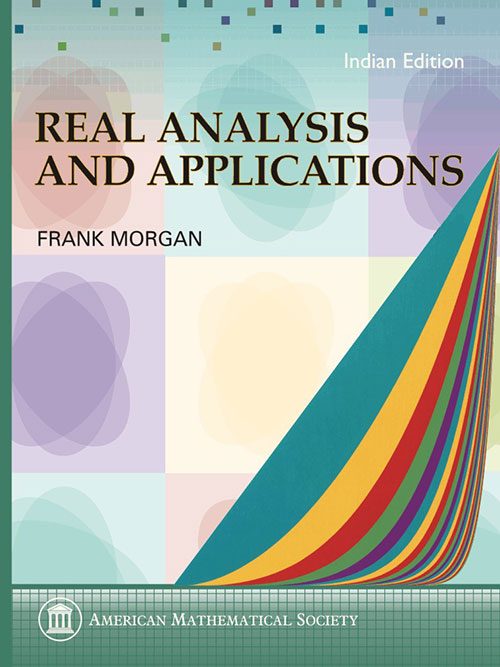Real Analysis and Applications: Including Fourier Series and the Calculus of Variations
Frank Morgan
Price
1320.00
ISBN
9780821891858
Language
English
Pages
208
Format
Paperback
Dimensions
180 x 240 mm
Year of Publishing
2012
Territorial Rights
Restricted
Imprint
American Mathematical Society
Catalogues

Real Analysis and Applications starts with a streamlined, but complete, approach to real analysis. It finishes with a wide variety of applications in Fourier series and the calculus of variations, including minimal surfaces, physics, economics, Riemannian geometry, and general relativity. The basic theory includes all the standard topics: limits of sequences, topology, compactness, the Cantor set and fractals, calculus with the Riemann integral, a chapter on the Lebesgue theory, sequences of functions, infinite series, and the exponential and Gamma functions. The applications conclude with a computation of the relativistic precession of Mercury''s orbit, which Einstein called "convincing proof of the correctness of the theory [of General Relativity]."

The text not only provides clear, logical proofs, but also shows the student how to derive them. The excellent exercises come with select solutions in the back. This is a text that makes it possible to do the full theory and significant applications in one semester. Frank Morgan is the author of six books and over one hundred articles on mathematics. He is an inaugural recipient of the Mathematical Association of America''s national Haimo award for excellence in teaching. With this applied version of his Real Analysis text, Morgan brings his famous direct style to the growing numbers of potential mathematics majors who want to see applications along with the theory. The book is suitable for undergraduates interested in real analysis.

Frank Morgan, Williams College, Williamstown, MA

Part I: Real numbers and limits

• Numbers and logic
• Infinity
• Sequences
• Subsequences
• Functions and limits
• Composition of functions

Part II: Topology

• Open and closed sets
• Compactness
• Existence of maximum
• Uniform continuity
• Connected sets and the intermediate value theorem
• The Cantor set and fractals

Part III: Calculus

• The derivative and the mean value theorem
• The Riemann integral
• The fundamental theorem of calculus
• Sequences of functions
• The Lebesgue theory
• Infinite series ?8n=1an
• Absolute convergence
• Power series
• The exponential function
• Volumes of n-balls and the gamma function

Part IV: Fourier series

• Fourier series
• Strings and springs
• Convergence of Fourier series

Part V: The calculus of variations

• Euler's equation
• First integrals and the Brachistochrone problem
• Geodesics and great circles
• Variational notation, higher order equations
• Harmonic functions
• Minimal surfaces
• Hamilton's action and Lagrange's equations
• Optimal economic strategies
• Utility of consumption
• Riemannian geometry
• Noneuclidean geometry
• General relativity
• Partial solutions to exercises
• Greek letters
• Index
THE BOOKPOINT (INDIA) PVT. LTD.